This optimization toolbox is an add-on/side project that I developed during optimization course at Georgia Tech. This toolbox is available for download in the downloads section. This toolbox can also serve as an educational tool to understand mainstream optimization techniques.

This tool includes an implementation of five gradient-based algorithms, including

• (1) Steepest Descend Algorithm (SDA),
• (2) Conjugate Gradient Algorithm (CGA),
• (3) Newton's Method,
• (4) Davidson-Fletcher-Powell (DFP) Method,
• (5) Broyden–Fletcher–Goldfarb–Shanno (BFGS)Method,
for multivariate function optimization. For two variable functions, a user-friendly interactive interface is available which portrays the optimization procedure step-by-step.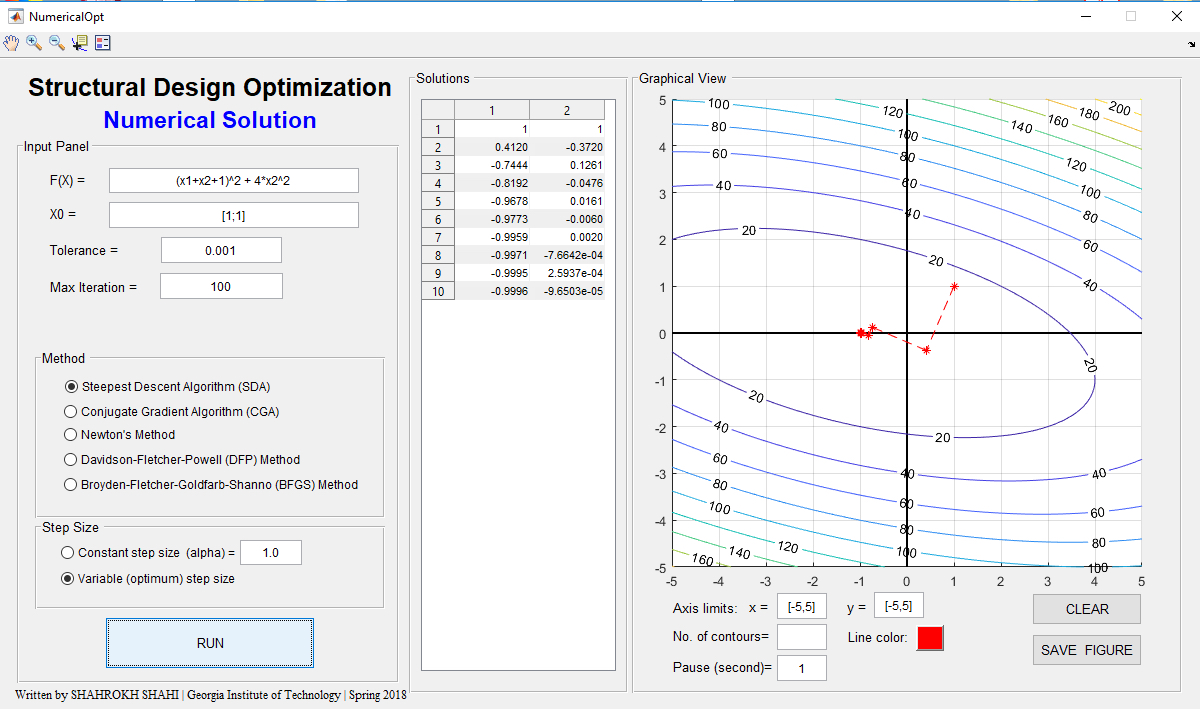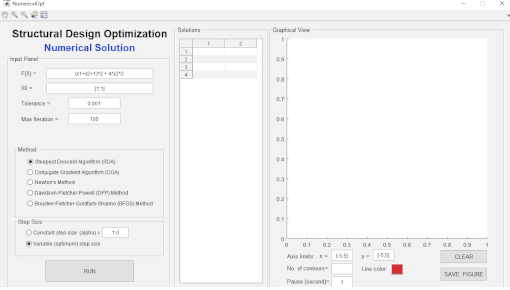Developed a MATLAB toolbox implementing optimization approaches for engineering applications.

Have you ever heard about golden ratio and it's applications (in fact, appearance) in the nature, physical processes, architecture, art, etc.? The golden-section search algorithm is another application of this mysterious number. This toolbox is an implementation of this optimization approach and provide an step-by-step visualization of the optimization process. This toolbox can also serve as an effective educational demo in the optimization courses.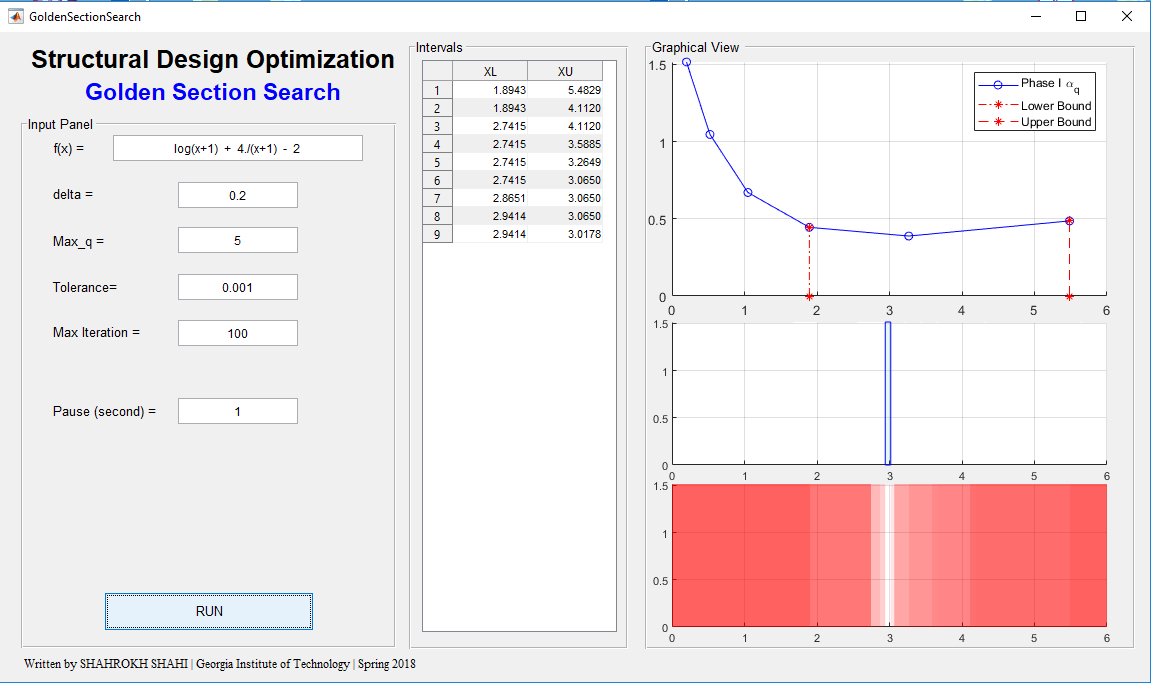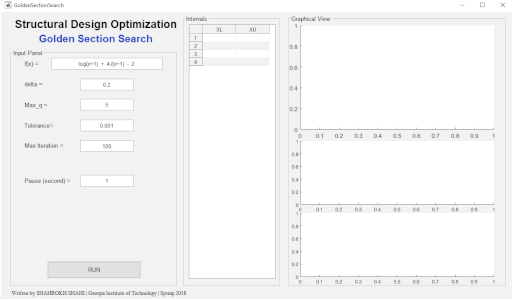Developed a MATLAB toolbox implementing optimization approaches for engineering applications.

#### (3) Constraint Optimization | Fitting Polygon Problem

This toolbox provides a demo of an interesting constraint optimization problem:
Find the coordinates of an n-sided polygon of greatest area, which will fit inside a given ellipse.
This was a challenging bonus problem in an optimization course at Georgia Tech, which the best record before this implementation could solve at most n=6, after more than an hour computation. However, this implementation was successfully tested for n<=20 with computational times less than a minute. Additionally, I also developed a simple graphical user interface to increase the flexibility of testing and visualization.

Within this toolbox, you can modify the ellipse equatio, also see the coordinates, Lagrange multipliers values, and a visualization of the optimum solution. This is also a visual proof of the fact that this problem has infinite solutions and each time new coordinates (and thus polygon) will be obtained, where all have the maximum area inside the peripheral ellipse.

This toolbox can also serve as an effective educational demo in the optimization courses.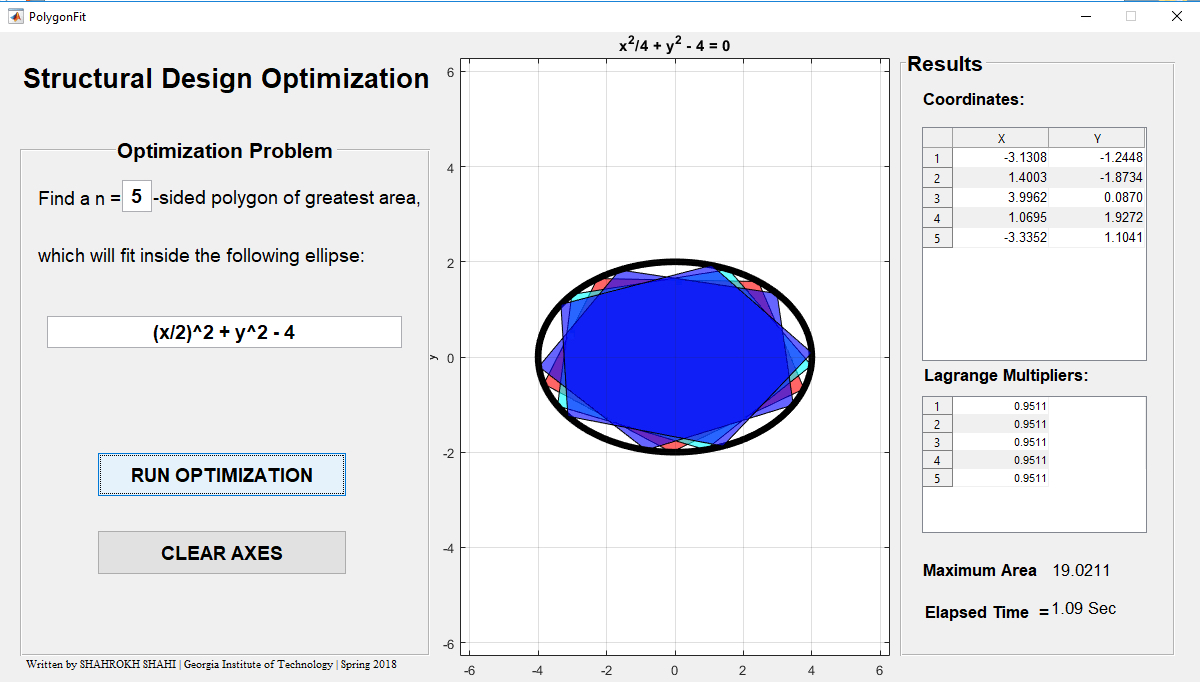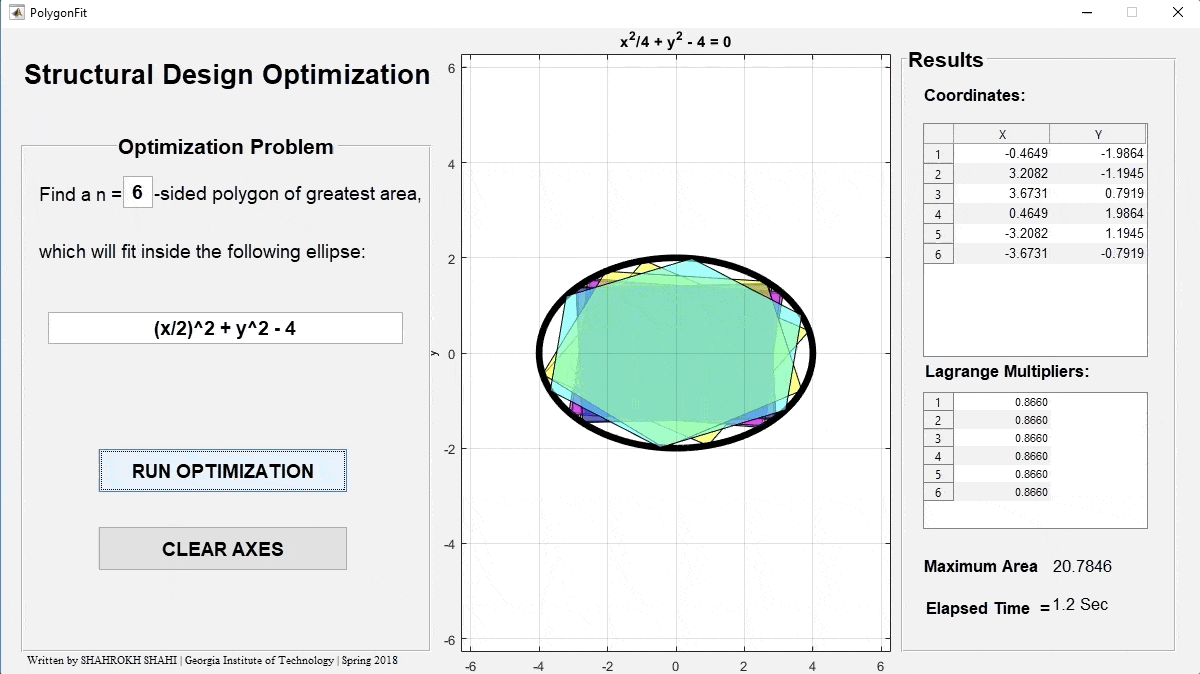Developed a MATLAB toolbox implementing optimization approaches for engineering applications.

#### (4) Constraint Optimization | Graphical Solution

Particularly, this toolbox can be used to solve the constraint optimization problems by visualizing the constraints over an arbitrary two variable function and obtaining the optimum value(s). The constraints can be in the form of equality, inequality and box constraints, and the user can easily define the functions and choose the colors to obtain a clear vision of the problem at hand. This toolbox can serve as an effective educational demo in the optimization courses.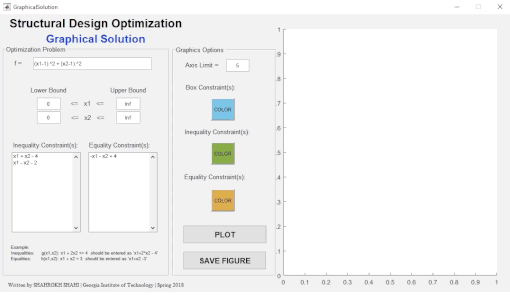Developed a MATLAB toolbox implementing optimization approaches for engineering applications.